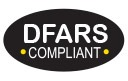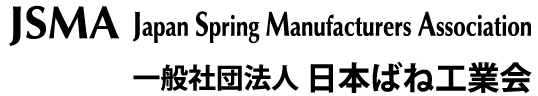New Alloy: Inconel® 617 is now available from Alloy Wire. Learn about Inconel® 617

# Designing a Round Wire Element

Where:

##### Example:

1.  To calculate the wire diameter and length required for a 750w/240v pencil bar fire element, operating at a maximum temperature of 1100°C, the total resistance of the element at operating temperature (Rt) will be:

2.  Using RW80 wire, the Temperature Resistance Factor (F) at 1100°C is 1.071 thus the total resistance of the element at 20°C (R) will be:

3.  Knowing the dimensions of the pencil bar, the length of wire that may be wound round it may be estimated (see coiled or spiral elements).  Thus, the resistance required per metre of wire will be:

For example a length of wire of 9 metres –

4.  RW80 of wire diameter 0.417mm has a resistance per metre of 7.91 ohms/m (see stock sizes page) which is closest to 7.97 ohms/m.

5.  To verify the actual wire length (L):

A change in wire length may mean adding or subtracting turns (altering the pitch of the wire) to achieve the total resistance value required.

This surface area loading should fall within the range shown in this table for a pencil bar fire element noting that a higher value gives a hotter element.  The surface area loading can be higher or lower if it is considered the heat transfer be better or worse, or depending upon the importance of the elements life.

If your calculated surface area loading is too high or low you should re-calculate changing one or more of the following:

• The wire length and diameter